# 7. ESonix Run Parameters¶

## 7.1. Regular Runs¶

The Regular Run Parameters are defined in three different places:

1. In the project settings (cf. Creating a project).
2. In the new run form (cf. Regular runs).
3. In a batch command file (cf. Batch Analysis).

The screen shots shown hereunder are visible from the “new project ” form, but the “new run” forms are almost the same.

The parameters shown by default in the new run form inherit from the one defined in the project form.

### Model Parameters¶

#### Units¶

units: Set of units in which the model and analysis results are given. SI (modified with bar instead of Pa) or Imperial.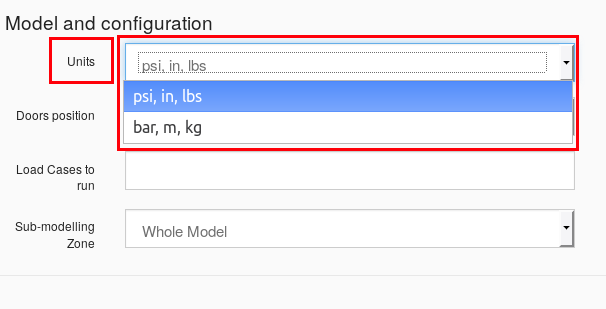Fig. 7.1 Units selection Combo Box

Batch Equivalence: unit_im

unit_im accepts a boolean (True or False) value.

specify True for an imperial units model:

unit_im = True


or False for a SI (bar instead of Pa) model:

unit_im = False


#### Doors Position¶

doors position: Set the initial position (configuration) of the
door to be used in the analysis.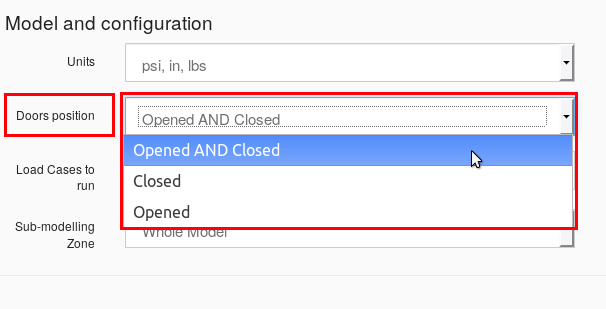Fig. 7.2 Doors Position selection Combo Box

Batch Equivalence: doors

doors accepts one of the strings: "open", "close" or "both".

Example:

doors = "close"  # to run only closed doors aircraft configuration


load cases to run: Comma separated list of load cases ID to run. This is useful to restrict calculations to a single or a subset of load cases as defined in the model spreadsheet. If blank, all load cases will be considered in the run.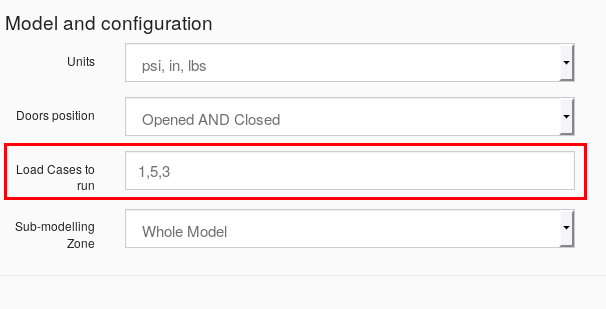Fig. 7.3 Load Cases to Run Text Box

Batch Equivalence: lcs

lcs accepts:

• empty (lcs =)
• None (lcs = None)
• a valid python list of integers (lcs = [1, 3, 5])

Example:

lcs = None  # Run all the load cases


or:

lcs = [1, 5, 3]  # Run load cases 1, 3 and 5


#### Sub-modelling Zone¶

sub-modelling zone: Set specific part of the model to be run. This is useful to restrict calculations to a single or a subset of model as defined in the model spreadsheet. If blank, the full model will be run.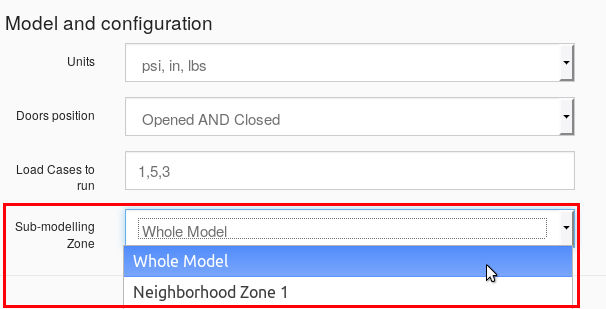Fig. 7.4 Sub-modelling Zone Combo Box

Batch Equivalence: submodel

submodel needs and integer (default to 0). If set to 0, then the whole model is analized. If set to 1, only the first neighbors zone. If set to 2, two rows of neighbours are accounted, etc.

Example:

submodel = 0  # Run the whole model


or:

submodel = 1  # Run the first "row" of neighbors.


### Runtime Parameters¶

#### Engine¶

engine: Thermodynamical engine to use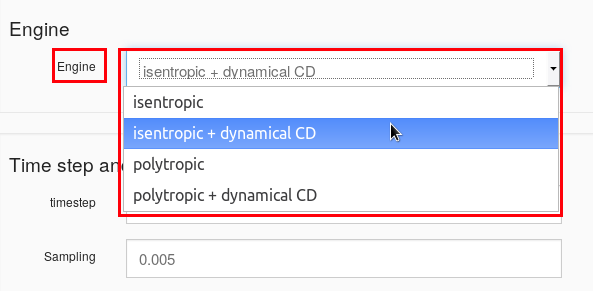Fig. 7.5 Engine Selection Combo Box

Batch Equivalence: engine

engine accepts one of the following strings:

• "isentropic": equivalent to the “isentropic” selection in the combo box.
• "isentropic_cddyn": equivalent to the “isentropic + dynamical CD” selection in the combo box.
• "polytropic": equivalent to the “polytropic” selection in the combo box.
• "polytropic_cddyn": equivalent to the “polytropic + dynamical CD” selection in the combo box.

Example:

engine = "isentropic_cddyn"  # "isentropic + dynamical CD" engine


#### Timestep¶

timestep: Simulation time increment.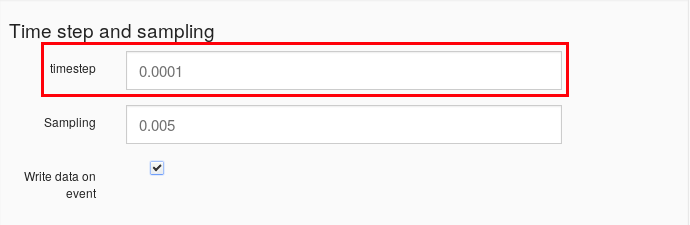Fig. 7.6 Timestep Text Box

Batch Equivalence: timestep

timestep accepts a float defining the time step to use.

Example:

timestep = 1e-5  # Time step = 0.00001


#### Sampling¶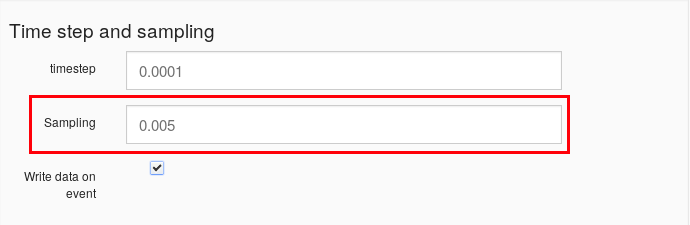Fig. 7.7 Sampling Text Box

sampling: Data sampling for disk storage.

Batch Equivalence: sampling

sampling accepts a float defining the sampling to use.

Example:

sampling = 1e-4  # sampling = 0.0001


#### Write Data on Event¶

Write data on event: When set to True, runtime records are written when an event occur. Should be let to True except to check compatibility with V2 results.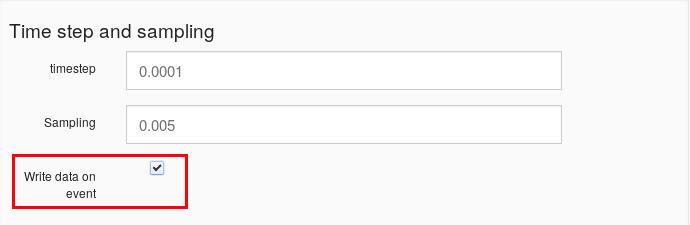Fig. 7.8 Write Data on Event Check Box

Batch Equivalence: write_on_event

write_on_event accepts a boolean value (True or False)

Example:

write_on_event = True


#### timeout¶

timeout: Simulation maximum time. If no other convergence criteria is met before, timeout stop the run.Fig. 7.9 Timeout Text Box

Batch Equivalence: timeout

timeout accepts a float defining the simulation timeout.

Example:

# Simulation will stop after 1.4s if no convergence is found:
timeout = 1.4


#### convergence criteria¶

convergence criteria: How to calculate convergence. This is a low-influence parameter as most of the time, timeout will end the calculation before the real convergence is reached.

As for now, three convergence criteria exist:

• H”: simulation stops once all differential pressure decrease.
• first_conv”: simulation stops once one volume reach ambient pressure.
• timeout”: simulation stops at timeout.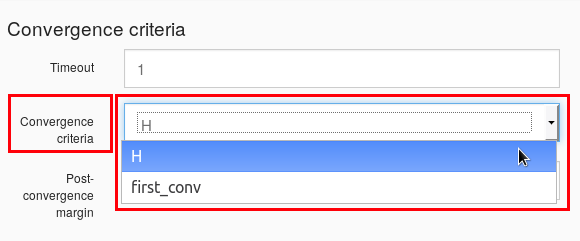Fig. 7.10 Convergence Criteria Combo Box

Batch Equivalence: convergence_criteria

convergence_criteria accepts one of “H”, “fisrt_conv” or “timeout” strings.

Example:

# Simulation will stop once all differential pressure decrease or after timeout no convergence is found:
convergence_criteria = "H"


#### Post-Convergence margin¶

post-convergence margin: Margin (in nb of steps) after convergence is
reached before stopping calculation.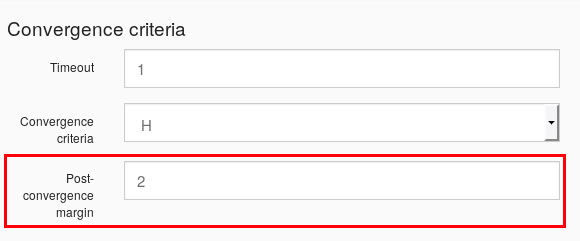Fig. 7.11 Post Convergence Margin Text Box

Batch Equivalence: pct_margin

pct_margin accepts a float.

Example:

# simulation will run additional 5% of elapsed time
# once convergence is met:
pct_margin = 5


adaptive strategy: Adaptive strategy to adopt for the run. The adaptive strategy is based on the courant number analysis. The proposed strategies are:
• no adaptive timestep”: legacy behavior, timestep is constant during the run.
• Courant Number flow control, no adaptive sampling”: Time step is dynamically adjusted during the run to match the adaptive strategy Max Co target. Sampling is as defined un Runtime tab.
• Courant Number flow control, time step on sampling cst ratio”: Time step is dynamically adjusted during the run to match the adaptive strategy Max Co target. Sampling is adjusted to keep constant the $${time\ step} / sampling$$ ratio.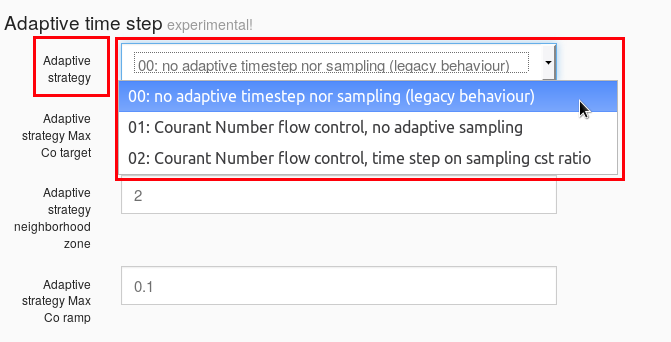Fig. 7.12 Adaptive Strategy Selection Combo Box

Batch Equivalence: adaptive

adaptive accepts an integer as per:

• 0: equivalent to “no adaptive timestep
• 1: equivalent to “Courant Number flow control, no adaptive sampling
• 2: equivalent to “Courant Number flow control, time step on sampling cst ratio

Example:

# adaptive time step, keeping initial timestep/sampling ratio:


#### adaptive strategy max co target¶

adaptive strategy max co target: Maximum Courant number allowed for an adaptive run. Defaulted to 1E-4. This target may be decreased for simple analysis. Decreasing the Courant Number target leads to more accurate yet more expensive runs.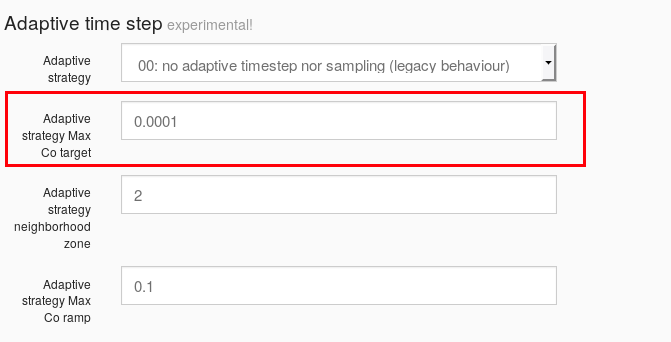Fig. 7.13 Adaptive Strategy Max CO Target Text Box

Batch Equivalence: adaptive_maxco

adaptive_maxco accepts a float.

Example:

# adaptive max CO Target is set to 1e-5


adaptive strategy neighborhood zone: Defines the neighborhood zone where Courant Number Criteria is checked. The greater this number, the more accurate yet expansive the calculation is. More details here.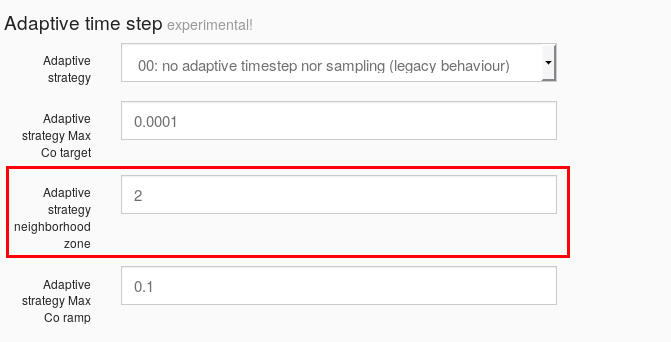Fig. 7.14 Adaptive Strategy Neighborhood Zone Text Box

Batch Equivalence: adaptive_zone

adaptive_zone accepts an integer.

Example:

# Check Courant number for one zone around the exploded volume


#### adaptive strategy max co ramp¶

adaptive strategy max co ramp: Defines how fast ESonix will reach the Courant Number Target. Use it for debugging only, as an incorrect number could lead to unstable results.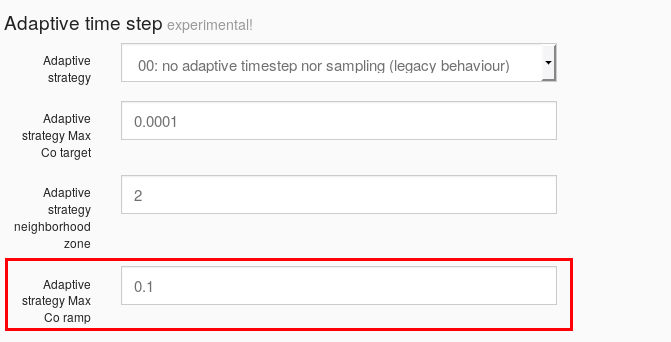Fig. 7.15 Adaptive Strategy Max CO Ramp Text Box

Batch Equivalence: adaptive_ramp

adaptive_ramp accepts a float<1.

Example:

adaptive_ramp = 0.1


#### default opening latency¶

default opening latency: Set a default latency for all opening feature.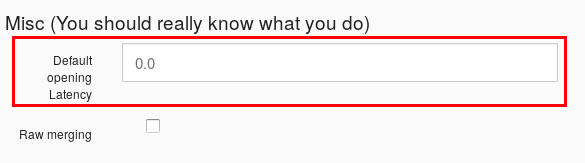Fig. 7.16 Default Opening Latency Text Box

Batch Equivalence: set_default_latency

set_default_latency accepts a float.

Example:

# set all the items to wait for 0.01sec before opening
set_default_latency = 0.01


#### raw merging¶

raw merging: Defaulted to False. When set to True (not advised), use the legacy behavior (V1) for merged load cases post-processing.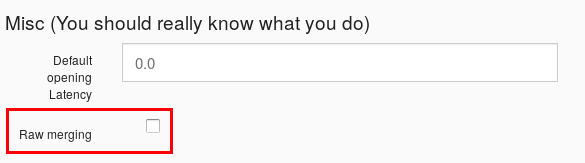Fig. 7.17 Raw Merging Check Box

Batch Equivalence: raw_merging

raw_merging accepts a boolean value (True or False).

Example:

raw_merging = False


## 7.2. Combination Runs¶

### Reference Node & “Nodes to combine”¶

Reference Node: Base node for combination. This node will keep it sown load cases numbering as it.

Nodes to combine: Selectable list of nodes to combine to Reference Node. If some of their load cases ID are common, the load-cases are mapped to available IDs.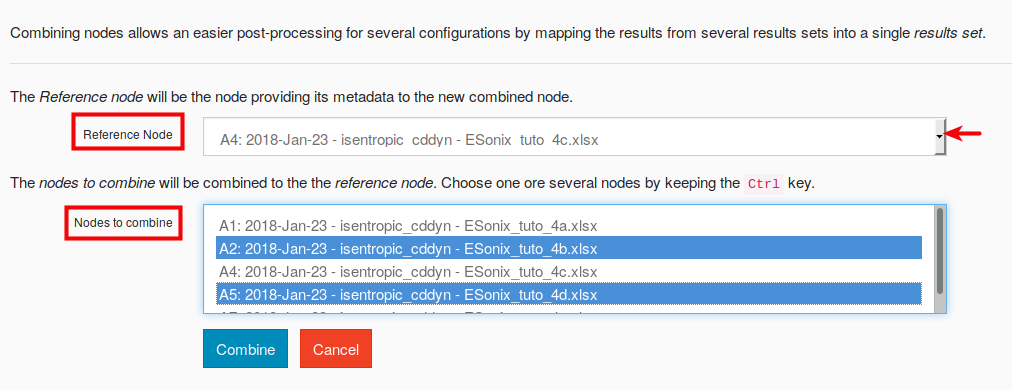Fig. 7.18 Combination Analysis Box

Batch Equivalence: nodes

nodes accepts a list of nodes to combine. The first node of the series will become the “reference node”. The nodes parameter therefore combine “Reference Node” and “Nodes to combine” into a single parameter.

Example:

# node A4 is the reference; A2 and A5 are combined with
nodes = [4, 2, 5]


## 7.3. Sensitivity Runs¶

Sensitivity: build a single set of results comparing results between existing nodes or between nodes (“reference” and/or “nodes to combine”) and a external file.

### “Reference Node” & “Nodes to combine”¶

Reference Node: Base node for sensitivity. This will be the first one on the bar plots.

Nodes to combine: Selectable list of nodes to compare.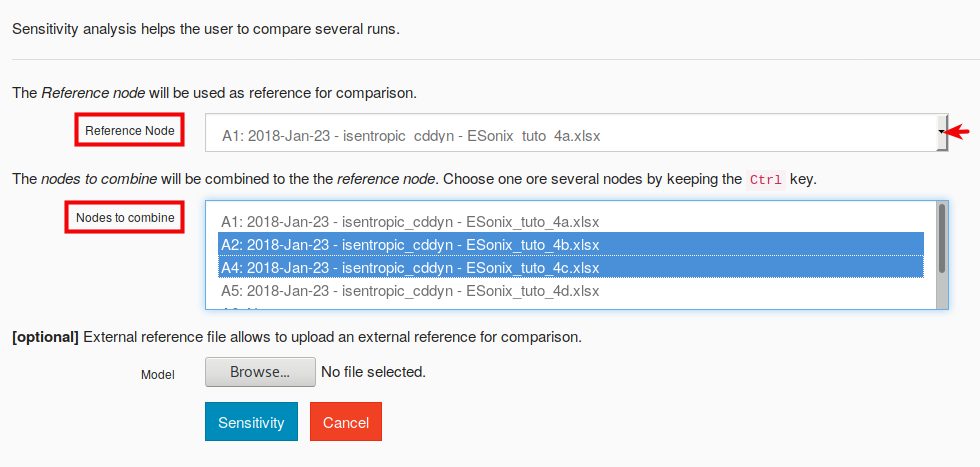Fig. 7.19 Sensitivity Analysis Box

Batch Equivalence: nodes

nodes accepts a list of nodes to compare. The first node of the series will become the “reference node”. The nodes parameter therefore compare “Reference Node” and “Nodes to combine” into a single parameter.

Example:

# node A1 is the reference; A2 and A4 are compared with
nodes = [1, 2, 4]


### “External reference file”¶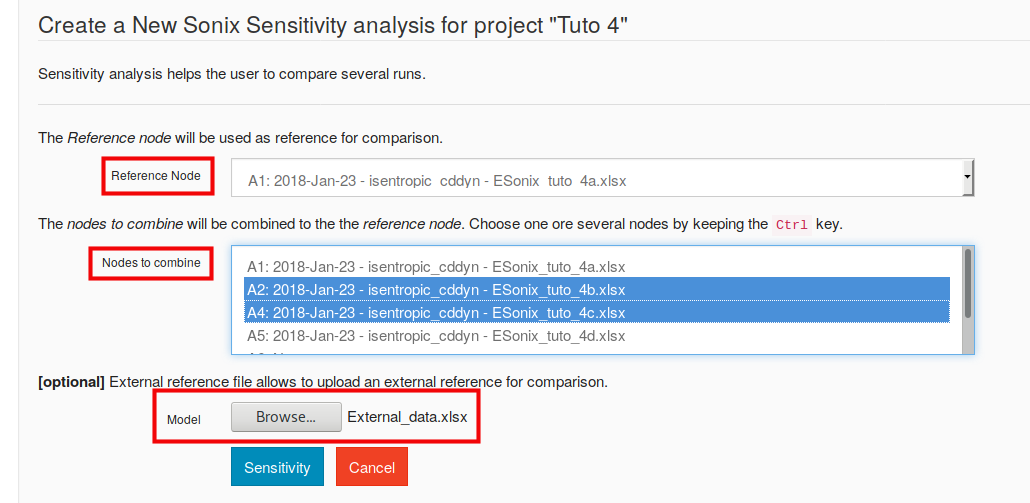Fig. 7.20 Sensitivity Analysis Box - External reference

The “External reference file” should be written in accordance to the following template: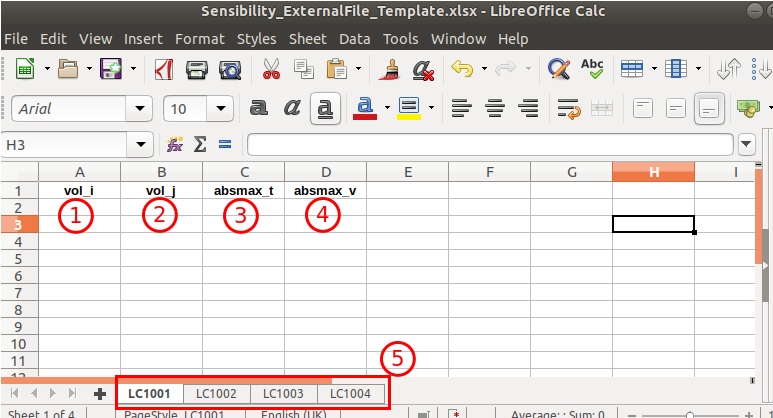Fig. 7.21 Sensitivity External File - Template

1. vol_i : volume identification (as per Esonix Model). Mandatory column with mandatory title.
2. vol_j : volume identification (as per Esonix Model). Mandatory column with mandatory title.
3. absmax_t : time when the maximum deltaP occurs. Mandatory column with mandatory title. Empty cells are accepted.
4. absmax_v : maximum deltaP. Negative values are accepted. Mandatory column with mandatory title.
5. Each sheet must be used for only one Load Case, which must be identified as per Esonix standard numeration LCXXXX.

Batch Equivalence: external_reference

external_reference accepts the external file name. The sensitivity will then compare the pre-defined nodes with the external_reference file into a single parameter.

Example:

external_reference = "External_data.xlsx"